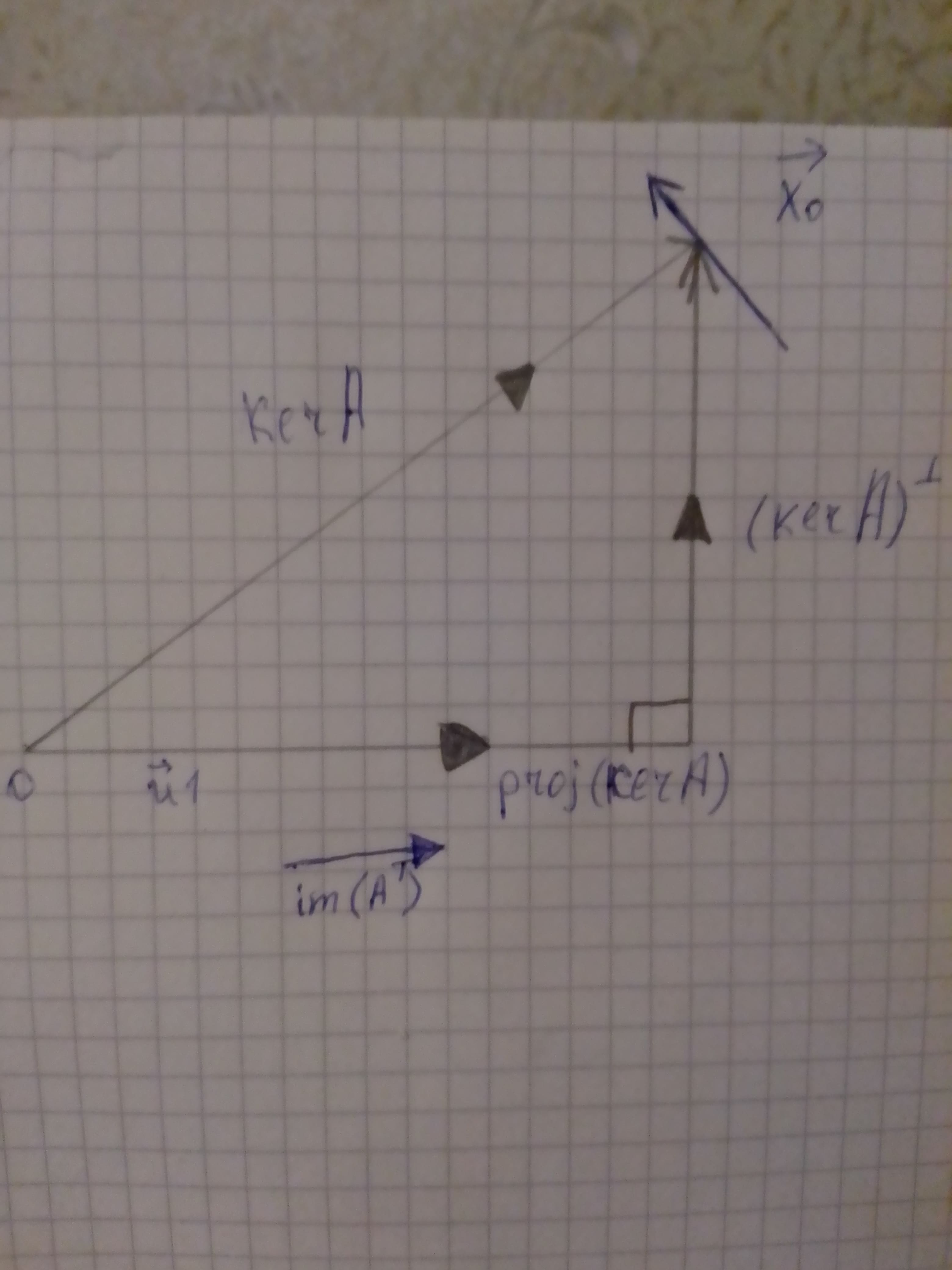# a) To graph: the ker(A),ker(A)^bot and im(A^T) ​ b) To find: the relationship between im(A^T) and ker(A).Brittney Lord 2021-02-27 Answered

a) To graph: the $ker\left(A\right),{\left(kerA\right)}^{\mathrm{\perp }}\phantom{\rule{1em}{0ex}}\text{and}\phantom{\rule{1em}{0ex}}im\left({A}^{T}\right)$
b) To find: the relationship between im $\left({A}^{T}\right)$ and ker (A).
c) To find: the relationship between ker(A) and solution set S
d) To find ${\stackrel{\to }{x}}_{0}$ at the intersection of $ker\left(A\right)\phantom{\rule{1em}{0ex}}\text{and}\phantom{\rule{1em}{0ex}}{\left(kerA\right)}^{\mathrm{\perp }}$
e) To find: the lengths of ${\stackrel{\to }{x}}_{0}$ compared to the other vectors in S

You can still ask an expert for help

• Questions are typically answered in as fast as 30 minutes

Solve your problem for the price of one coffee

• Math expert for every subject
• Pay only if we can solve itCorben Pittman

a) Given:
The image of linear transformation $T\left(\stackrel{―}{x}\right)=A\left(\stackrel{―}{x}\right)$ is the span of the column vectors of A.
The kernel of a linear transformation $T\left(\stackrel{―}{x}\right)=A\left(\stackrel{―}{x}\right)\mathfrak{o}m{\mathbb{\text{R}}}^{m}\to {\mathbb{\text{R}}}^{n}$ consists of all zeros of the transformation.
That is the solution of the equation $T\left(\stackrel{―}{x}\right)=A\left(\stackrel{―}{x}\right)=0.$
We denote the kernel of T by ker (A) or ker (T) and it is the solution set of the linear system $A\left(\stackrel{―}{x}\right)=0.$
Graph:Interpretation:
Consider the linear system $A\left(\stackrel{\to }{x}\right)=\stackrel{\to }{b}f\phantom{\rule{1em}{0ex}}\text{or}\phantom{\rule{1em}{0ex}}A=\left[\begin{array}{cc}1& 3\\ 2& 6\end{array}\right]\phantom{\rule{1em}{0ex}}\text{and}\phantom{\rule{1em}{0ex}}\stackrel{\to }{b}=\left[\begin{array}{c}10\\ 20\end{array}\right].$
$A\left(\stackrel{\to }{x}\right)=\stackrel{\to }{0}$
$\left[\begin{array}{cc}1& 3\\ 2& 6\end{array}\right]\left[\begin{array}{c}{x}_{1}\\ {x}_{2}\end{array}\right]=0$
${x}_{1}=-3t,{x}_{2}=t$
$t=parametr$
$\left[\begin{array}{c}{x}_{1}\\ {x}_{2}\end{array}\right]=\left[\begin{array}{c}-3t\\ t\end{array}\right]=\left[\begin{array}{c}-3\\ 1\end{array}\right]t$
Thus, ker (A) is the line spanned by $\left[\begin{array}{c}-3\\ 1\end{array}\right]\in {\mathbb{\text{R}}}^{2}.$
Consider im (A) which is spanned by ${\stackrel{\to }{u}}_{1}=\left[\begin{array}{c}1\\ 2\end{array}\right].$ Then,
${\left(kerA\right)}^{\mathrm{\perp }}=ker\left(A\right)-proj\left(kerA\right)$
${\left(kerA\right)}^{\mathrm{\perp }}=ker\left(A\right)-\left({\stackrel{\to }{u}}_{1}.kerA\right){\stackrel{\to }{u}}_{1}$
${\left(kerA\right)}^{\mathrm{\perp }}=\left[\begin{array}{c}-3\\ 1\end{array}\right]-\left[\left[\begin{array}{c}1\\ 2\end{array}\right]\cdot \left[\begin{array}{c}-3\\ 1\end{array}\right]\right]\left[\begin{array}{c}1\\ 2\end{array}\right]$
${\left(kerA\right)}^{\mathrm{\perp }}=\left[\begin{array}{c}-3\\ 1\end{array}\right]+\left[\begin{array}{c}1\\ 2\end{array}\right]$
${\left(kerA\right)}^{\mathrm{\perp }}=\left[\begin{array}{c}-2\\ 3\end{array}\right]$
Consider ${A}^{T}=\left[\begin{array}{cc}1& 2\\ 3& 6\end{array}\right].$
Since